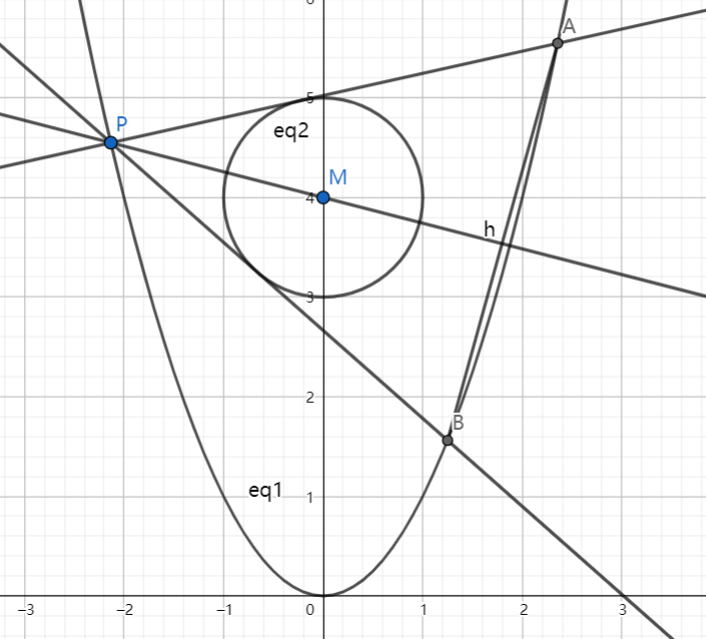# SAT1000 - P802

Geometry Level pendingAs shown above, the parabola $C_1: x^2=y$, circle $C_2: x^2+{(y-4)}^2=1$, and $M$ is the center of circle $C_2$.

Point $P$ is a point on $C_1$ (not at $(0,0)$), and $l_1, l_2$ are two lines tangent to $C_2$ and they intersects with $C_1$ at point $A,B$ respectively. Line $l$ passes through $M$ and $P$.

If $l \perp AB$, find the equation of line $l$.

The equation can be expressed as: $y=\pm k x+b\ (k>0)$ . Submit $\lfloor 1000(k+b) \rfloor$.

Have a look at my problem set: SAT 1000 problems

×